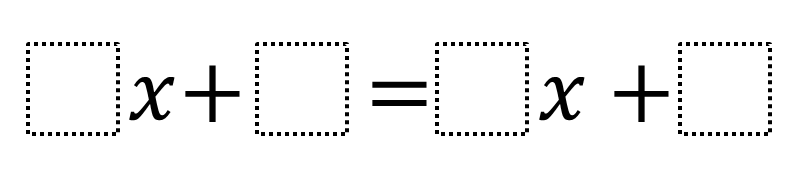Home > Grade 8 > Expressions & Equations > Create an Equation with a Given Solution

# Create an Equation with a Given Solution

Directions: Using the digits 0 to 9 at most one time each, fill in the boxes to write three equations whose solution is -1/2.### Hint

What does it mean to be a solution of the equation?
How would you check if a value is a solution to an equation?
What kind of numbers would you put in for the coefficients of x?